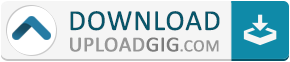#Do not remember me!

### Categories» » » Practical Geometry Algorithms: with C++ Code

## Practical Geometry Algorithms: with C++ CodeEnglish | 2021 | ASIN : B099XZNJ2R | 193 pages | PDF | 7.05 MB

This book presents practical geometry algorithms with computationally fast C++ code implementations. It covers algorithms for fundamental geometric objects, such as points, lines, rays, segments, triangles, polygons, and planes. These algorithms determine the basic 2D and 3D properties, such as area, distance, inclusion, and intersections. There are also algorithms to compute bounding containers for these objects, including a fast bounding ball, various convex hull algorithms, as well as polygon extreme points and tangents. And there is a fast algorithm for polyline simplification using decimation that works in any dimension.

These algorithms have been used in practice for several decades. They are robust, easy to understand, code, and maintain. And they execute very rapidly in practice, not just in theory. For example, the winding number point in polygon inclusion test, first developed by the author in 2000, is the fastest inclusion algorithm known, and works correctly even for non-simple polygons. Also, there is a fast implementation of the Melkman algorithm for the convex hull of a simple polyline. And much more.Name:* E-Mail: Security Code: *